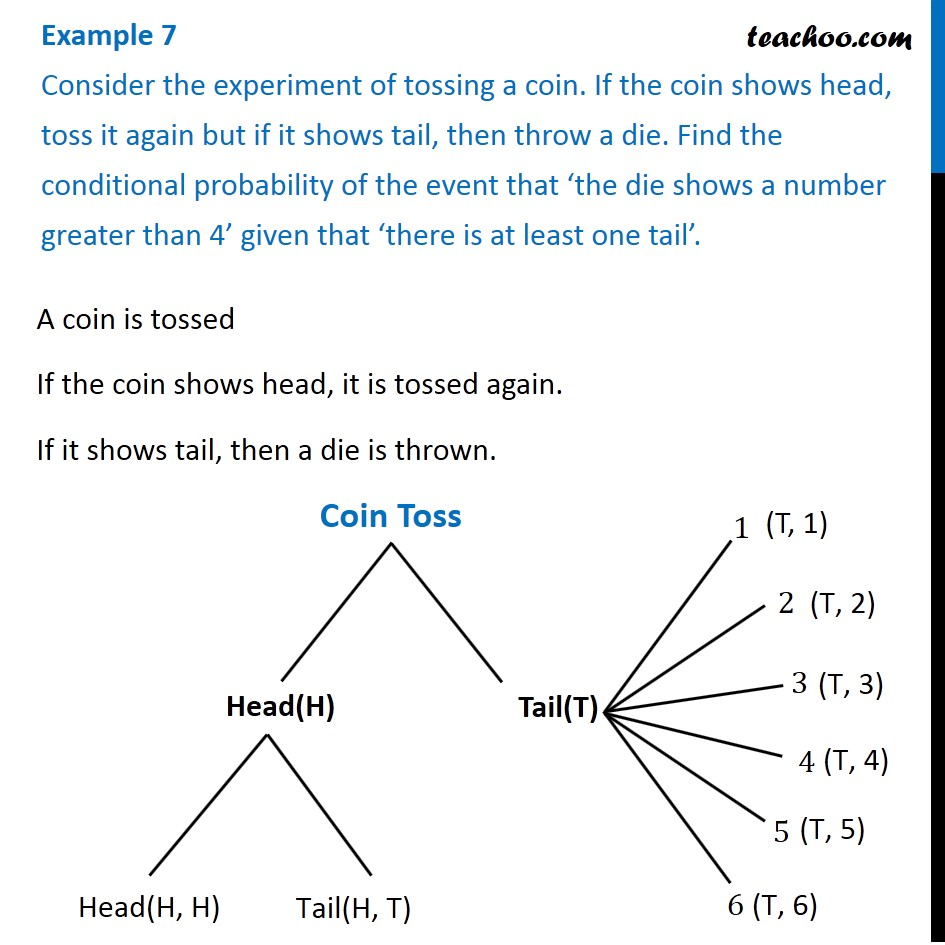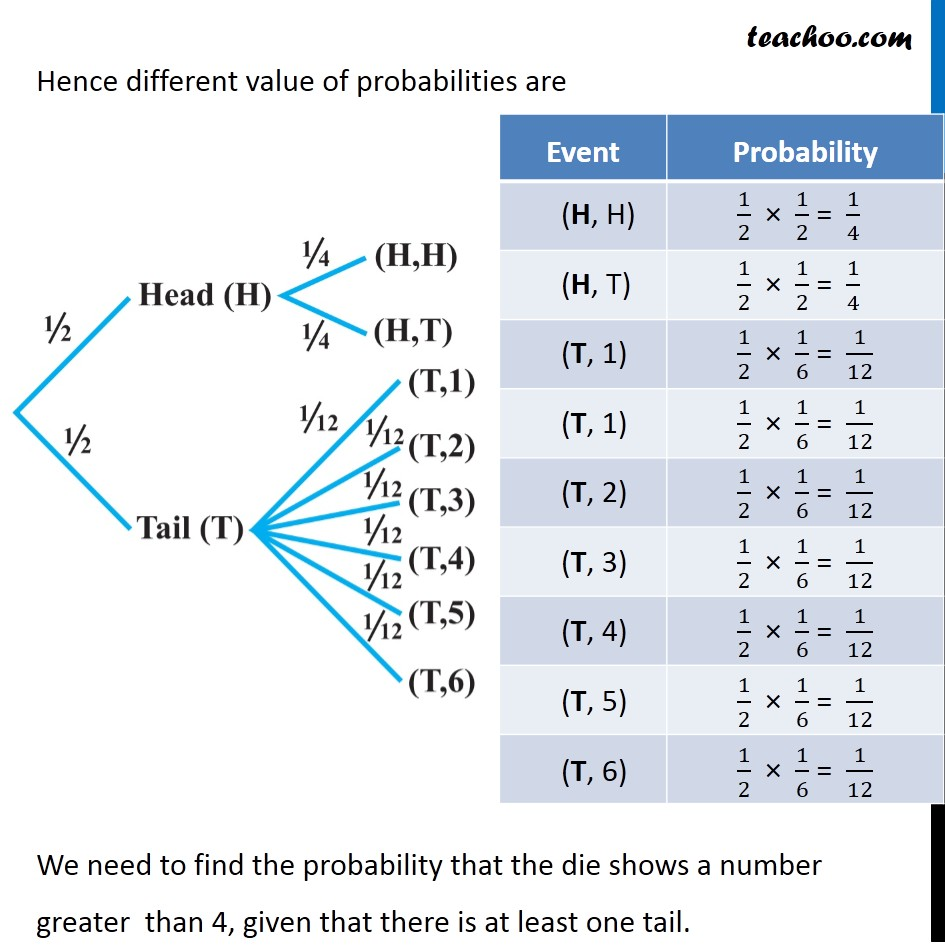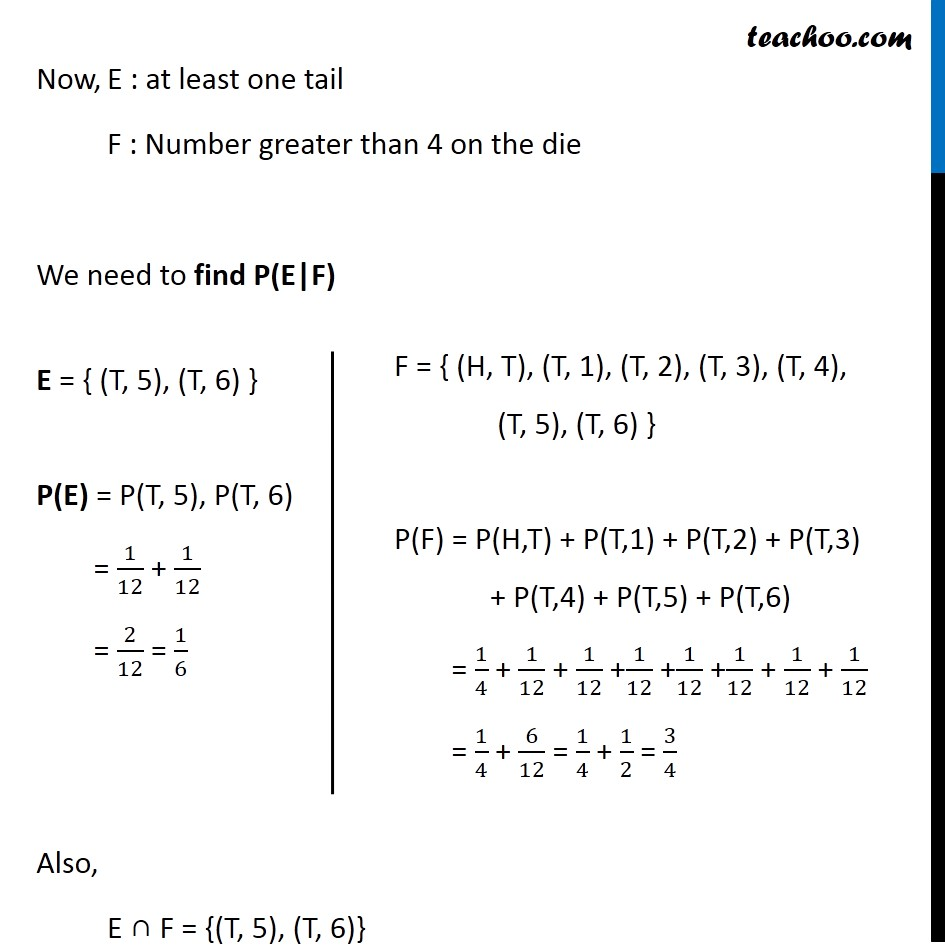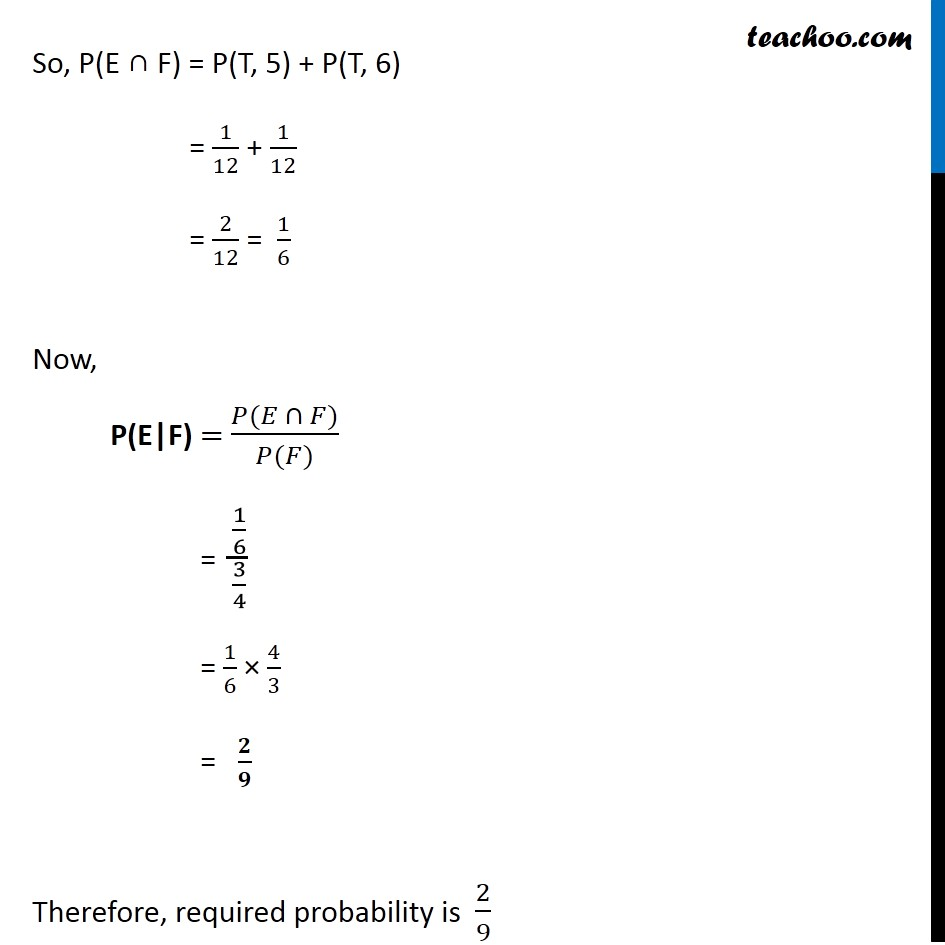Chapter 13 Class 12 Probability

Class 12
Important Questions for exams Class 12Solve all your doubts with Teachoo Black (new monthly pack available now!)

### Transcript

Example 7 Consider the experiment of tossing a coin. If the coin shows head, toss it again but if it shows tail, then throw a die. Find the conditional probability of the event that ‘the die shows a number greater than 4’ given that ‘there is at least one tail’.A coin is tossed If the coin shows head, it is tossed again. If it shows tail, then a die is thrown. Hence different value of probabilities are We need to find the probability that the die shows a number greater than 4, given that there is at least one tail. Now, E : at least one tail F : Number greater than 4 on the die We need to find P(E|F) Also, E ∩ F = {(T, 5), (T, 6)} E = { (T, 5), (T, 6) } P(E) = P(T, 5), P(T, 6) = 1/12 + 1/12 = 2/12 = 1/6 F = { (H, T), (T, 1), (T, 2), (T, 3), (T, 4), (T, 5), (T, 6) } P(F) = P(H,T) + P(T,1) + P(T,2) + P(T,3) + P(T,4) + P(T,5) + P(T,6) = 1/4 + 1/12 + 1/12 +1/12 +1/12 +1/12 + 1/12 + 1/12 = 1/4 + 6/12 = 1/4 + 1/2 = 3/4 So, P(E ∩ F) = P(T, 5) + P(T, 6) = 1/12 + 1/12 = 2/12 = 1/6 Now, P(E|F) = (𝑃(𝐸 ∩ 𝐹))/(𝑃(𝐹)) = (1/6)/(3/4) = 1/6 × 4/3 = 𝟐/𝟗 Therefore, required probability is 2/9